# D'Alembert operator

(diff) ← Older revision | Latest revision (diff) | Newer revision → (diff)

wave operator, d'Alembertian

The second-order differential operator which in Cartesian coordinates assumes the following form: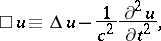whereis the Laplace operator andis a constant. Its form in spherical coordinates is: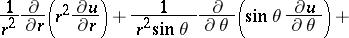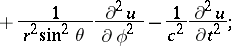in cylindrical coordinates: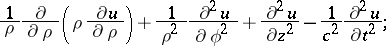in general curvilinear coordinates: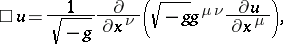whereis the determinant of the matrix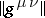formed from the coefficients of the metric tensor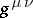.

Named after J. d'Alembert (1747), who considered its simplest form when solving the one-dimensional wave equation.

In the last equation above, the Einstein (summation) convention applies to the right-hand side (i.e. there is a summation involved over all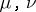).